[BACK]
 Intelligent Automation & Soft ComputingDOI:10.32604/iasc.2021.015461Article

Analysis of Iterative Process for Nauru Voting System

1Shaheed Benazir Bhutto Women University Peshawar Pakistan
2Athlone Institute of Technology Athlone, Ireland
*Corresponding Author: Neelam Gohar. Email: neelam.gohar@sbbwu.edu.pk
Received: 23 November 2020; Accepted: 29 January 2021

Abstract: Game theory is a popular area of artificial intelligence in which the voter acknowledges his own desires and favors the person he wants to be his representative. In multi-agent systems, social choice functions help aggregate agents’ different preferences over alternatives into a single choice. Since all voting rules are susceptible to manipulation, the analysis of elections is complicated by the possibility of voter manipulation attempts. One approach to understanding elections is to treat them as an iterative process and see if we can reach an equilibrium point. Meir et al. proposed an iterative process to reach a stable outcome, i.e., Nash Equilibrium. This technique, explored in previous work, converges to a Nash equilibrium for plurality voting, along with a tie-breaking rule that chooses a winner according to a linear order of preferences over candidates. Almost all the scoring rules have been studied in previous work, we identified the iterative processes of the Nauru voting system. We analyzed the Nauru voting system with Copelands and lexicographic rule for tiebreaking. Nauru is the modified version of Borda counting. Like Borda counting, Nauru voting system scores each candidate with different points. In the iterative behavior analysis of the Nauru voting system, when two or more winning candidates have the same score, a tie occurs. To break the tie, we use the Copeland method, which is a pairwise comparison to rank the candidates. If there is still a tie, we break it using the traditional linear ordering method, the lexicographic rule. We have observed cycles for different manipulative moves.

Keywords: Multi agent system (MAS); iterative voting; manipulation; game theory; group decision making

1  Introduction

For decision making in groups, voting system has been used as a tool for centuries . Voting and aggregation mechanisms have also been used for other tasks such as aggregating search results from the Web  and collaborative filtering .

Social choice is concerned with the analysis and design of methods for aggregating the preferences of multiple agents and is considered a fundamental tool for the study of multi-agent systems. A multi-agent system requires a mechanism to aggregate opinions for a joint decision. One such mechanism is a voting system in which each voter ranks several options (such as people, common plans, rooms for a meeting, etc.). The result is in the form of an option using a voting rule after aggregating the voters’ preferences over the available options. Sometimes voters misstate their preference order to make the outcome more favorable to them; such type of voting is called strategic or tactical voting.

In social choice theory, the important result of Gibbard-Satterthwaite Theorem [6,7] states that any non-dictatorial “reasonable” voting rule is susceptible to manipulation, so developing voting models for truthful voters is pointless. Effective manipulation is related to the knowledge that agents have about the true preferences of other voters. Much work is based on complete information.

One way to understand the electoral process is to look at the dynamics of manipulation to reach an equilibrium state where voters are satisfied with their manipulations and no voter wants to switch preferences. The most stable solution is Nash Equilibrium. It can be used as a tool for analyzing the election process. Such an approach has been defined in terms of a formal model of iterative voting by Meir et al. , their work can be considered as a decision process where voters can change their vote at any time (just like in committees or online facilities). A similar process can be seen on various websites to coordinate dates of an event such as www.doodle.com; after casting an initial vote, each participant is allowed to change their vote. Players change their choices one at a time; the iterative voting process is better suited for a small number of agents or when a choice is very close.

Meir et al.  showed that under the plurality rule with deterministic tie-breaking, voters must converge to a Nash equilibrium when choosing the best available answers in a given state. However, convergence for better answers is not guaranteed under the setting with weighted voters.

Deterministic tie-breaking rule plays an important role in the convergence of equilibria, under positional scoring rules, convergence is not guaranteed unless we restrict the choice of tie-breaking rule. In the current paper, we investigate the non-convergence of the Nauru voting system with deterministic tie-breaking rule in the context of iterative voting for the Nauru voting system.

The paper studied the multiprocessor machine scheduling problems in the context of coordination mechanisms. The properties of the pure strategy Nash Equilibrium were considered for the selfish scheduling games. The system converges to a pure strategy Nash Equilibrium in linear time, the convergence rate is computed by iteratively playing the jobs their best responses starting from an arbitrary state. This is important for the price of anarchy and upper and lower bounds on the price of anarchy have also been derived .

Voters have incomplete information about the preference of other voters, they only know the score of all candidates to change their vote. Iterative processes that converge to a steady state or generate cycles are often needed to analyze the dynamics. Our contribution is the game theoretic analysis of the Nauru voting system with various manipulative moves in weighted and unweighted settings.

1.1 Contribution

We study iterative voting for the Nauru voting rule in a simple form for a given set of moves, and our results are for the set of manipulations where the process leads to a cycle. We find that even in this simple model, the dynamics for the Nauru voting rule do not converge. This is the first time this iterative process for Nauru has been analyzed, and we take into account that voters have incomplete information about the preferences of other voters. Our results show that voters can assume declared or truthful preferences and the process does not converge for a given set of manipulations.

2  Related Work

The formal model we use is based on the iterative model presented by Meir et al. . The notion of iterative voting and the existence of equilibria for manipulation dynamics emerge from previous literature. Voting systems and convergence to equilibria have been studied in computer science [8,1013]. In group decision making, an iterative process to reach a stable outcome was operated for agents , but the method used was “value like money” among agents, thus not relevant to a voting system. Airiau et al.  analyzed the possibility of equilibrium in such an iterative process by using voting rules such as plurality.

Achieving equilibrium with an iterative process has been considered by previous researchers, but mainly when deciding on an allocation of public goods. A summary of most of the work can be found in the work of Laﬀont , however the preferences chosen are single peaked preferences. Feddersen et al.  also restrict their search for equilibria to single peaked preferences. A more diﬀerent approach was taken by Messner and Polborn , analyzing equilibria through coalitional manipulation.

Most of the above work assumed that voters have some knowledge about the preferences of the other players, and this is one of the main limitations of their work.

In terms of knowledge of other players’ preferences, Chopra et al.  showed the results by restricting a player’s knowledge of other players’ preferences, they analyzed the iterative process for plurality voting rule. A model was proposed by Myerson and Weber , it is assumed that voters have some knowledge about the winning candidate (e.g., based on pre-election polls), a Nash equilibrium for scoring rules was found, but not every election leads to an equilibrium. The limitations of the iterative method and the importance of the equality rule were considered by Lev et al.  and it was proved that the use of a constrained equality rule, such as linear order, does not by itself guarantee convergence. The work of Hwang, Ching Lai et al. . has given an overview of group decision making under multiple criteria, existing methods, properties and applicability in the complexity of group decision making. In , the authors presented a multi-agent system for resource allocation and process scheduling in which agents communicate and cooperate with each other to produce an optimal schedule.

Group decisions were explored in terms of voting properties, which provides well formalized techniques for preference aggregation. Voting also provides some efficient algorithms for resolving disagreements among participants. There are many works on game-theoretic analysis of voting that consider voters as strategic agents and reach Nash Equilibrium as a solution concept for preference aggregation scenarios. The iterative voting model introduced by Meir et al.  is used for non-convergence of Nauru voting system. Another framework adopted by researchers is adding truth biasness to games proposed by Thompson et al. . Truth biasness means that voters are truthful because they cannot influence the election outcome.

3  Preliminaries

Symbols and notations used in the analysis of Nauru Voting System are discussed here. Some of the notations and definitions are taken from [8,10,12].

3.1 Nauru Scoring System

Nauru is a position scoring rule. Voters give different points to candidates, such as (A1, B1/2, C1/3, Z.1/n) A1 means that the voter gives 1 point to the first candidate, B1/2 means that the voter gives 0.50 points to the second candidate, and so on.

3.2 Preferences

Voters’ preferences are the ranking of candidates according to their choice. The two types of voter preferences are described below.

3.3 True/Fixed Preference

True preference is the actual ranking of candidates according to their true choice. True preferences are fixed, voters do not change them and want their favorite candidate to win the election.

3.4 Declared/Stated Preference

In declared preference, voters change their true preference depending on the situation and circumstances. Declared preferences are associated with a particular state.

3.5 Switching

Switching is the mechanism by which voters change their preference to obtain a desirable candidate or a favorable election outcome.

3.6 Notation for Ranking

Voters rank candidates by using notation such as“ ”. A B C means that A is the first choice, B is the second choice and so on.

3.7 Transition

Transition is the mechanism by which the voter changes his vote and the transition is from one state to another state. We allow one voter at a time to change his preference list.

3.8 Manipulative Move

Manipulative move is the rational move where the voter changes his preference in support of a preferred candidate to win the election. There are the different moves defined in [10,12].

The following election rules are used a. Borda election rule b. Nauru scoring system. Copeland method and lexicographic rule are used to resolve ties.

3.9 Borda Rule

In Borda rule, 0 point is assigned to the last election and 1 point to the penultimate election . Borda ranks each candidate in whole numbers, starting with the least preferred candidate. The points of each candidate are added and the candidate with the highest score is the winner of the election .

3.10 Example 1

Table 1: Borda Rules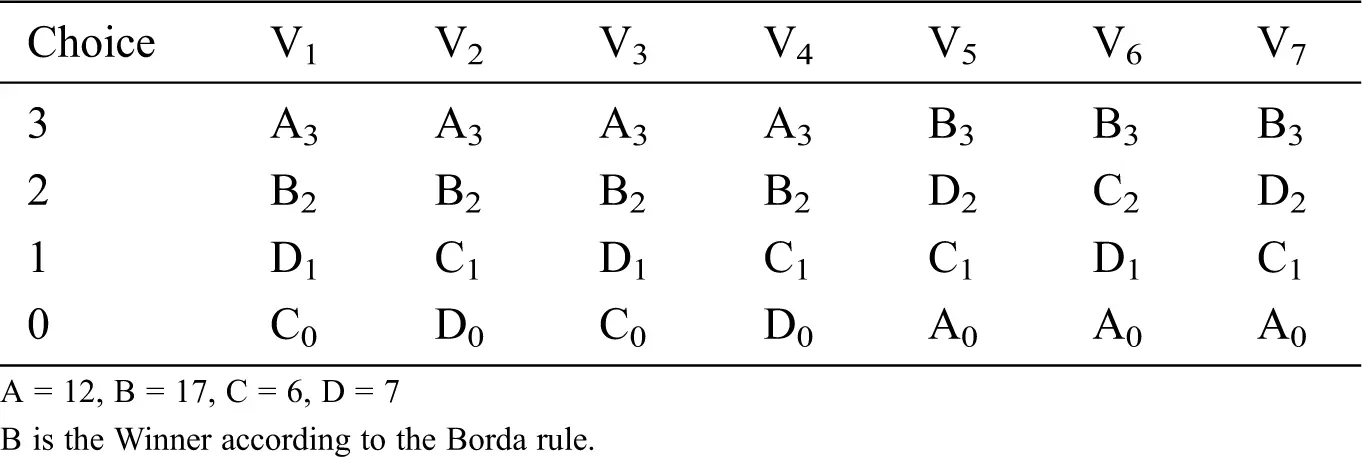3.11 Nauru Scoring System

Nauru, the modified form of the Borda rule, awards 1 point for the first choice, 1/2 point for the second choice, 1/3 for the third choice, 1/ 4 for the fourth choice, 1/5 for the fifth choice, etc. In the Nauru scoring system, the score is derived by assigning points to the various positions in a predetermined ranking order. Thus, the points of each candidate are added together and the candidate with the highest score is the winner . The Borda voting rule ranks candidates in whole numbers and assigns zero to the least preferred candidate, but in Nauru every candidate is ranked in a decimal point system except the first, hence it is sometimes called the decimal point system. The following example shows how the Nauru electoral system works.

3.12 Example 2

No of Candidates = 03 No of Voters = 04 Candidates = A, B and C Voter’s = V1, V2, V3, V4

Voting rule is Nauru point system and voters start from their declared Preferences.

Table 2: Nauru point System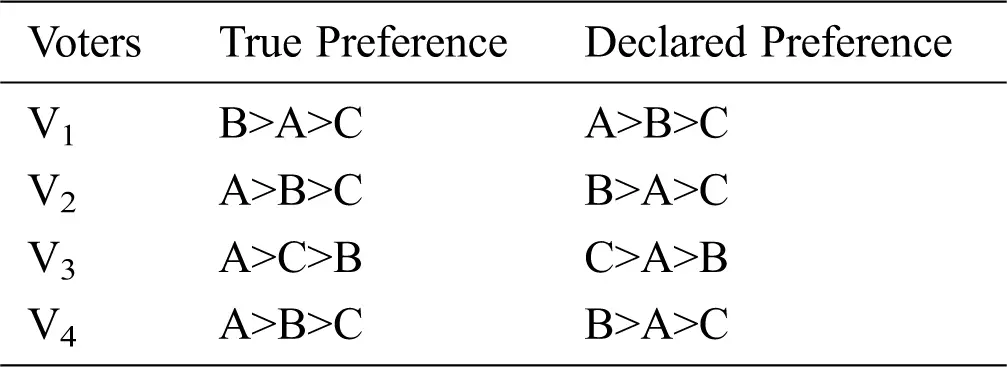Table 2a: State = 0Candidate B has maximum points so B is the winner of the election.

3.13 Tie Breaking Rules

During the election, when two candidates receive the same points, a tie occurs between the candidates. To break the tie, we use two methods, the Copeland method and the lexicographic rule. The Copeland method is a pairwise comparison where one candidate is compared to every other candidate. In , we use the Copeland method as the tie-breaking rule because it performs a comparison between candidates. A candidate beats every other candidate in the comparison and selects the best one . Copeland method can select a more desirable winner than just following the linear order.

3.14 Example 3

Table 3: Copeland’s Method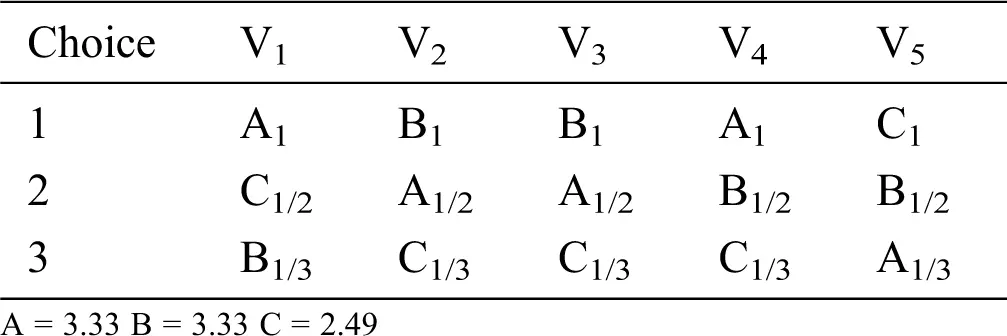In above example we have three candidates A, B and C and tie occur between candidates A and B, so we use the pair wise comparison rule (Copeland’s method) to break the tie between the candidates. In three candidate case the comparison is A.B, A.C, B.C.

We compare A.B in the above table V1 prefers candidate A over B, so candidate A gets 1 point V2 prefers candidate B over A, so candidate B gets 1 point.

A.B A = 2 B = 3 Result: B = 1

If tie occurs between A and B, then each candidate receives 0.5.

A.C   A = 1 + 1 + 1 + 1 = 4  C = 1      Result: A = 1

B.C   B = 1 + 1 + 1 = 3    C = 1 + 1 = 2  Result: B = 1

A = 1   B = 1 + 1 = 2      C = 0

If the tie is not broken by the Copeland method, then we break the tie by the lexicographic rule. The lexicographic method is the traditional tie-breaking rule and selects candidates according to linear order.  In lexicographic rules, the voting systems are not dictatorial. These classical methods are most commonly used as tie-breaking rules in plurality voting systems. [10,26]

3.15 Example 4

Table 4: Lexicographical methodLet there are three candidates and four voters.

Table 4a: State = 0If there is a tie between candidates A and B, we use the Copeland method; if there is another tie between candidates A and B, we use the lexicographic rule.

3.16 Weighted Voting System

In a weighted voting system, weights are assigned to the voters. Each participant/voter has different weights. Weighted system means that some preferences have more weight than the preferences of the other voters. Voters have equal influence on the outcome of an election. Voter weights can represent a group of like-minded voters who have the same preference. The weights remain the same throughout the election. In the Nauru electoral system, an individual voter can hardly have a significant impact on the election outcome, so we assign a weight to voters that is in real positive numbers so that individual weighted voters can change the election outcome more effectively and efficiently than unweighted voters. For weighted Nauru voters, we multiply the Nauru numbers by the weight of the voters, which are converted to floating point numbers. The following example shows how the Nauru voting system works in a weighted setting.

3.17 Example 5

Table 5: Nauru Weighted Point System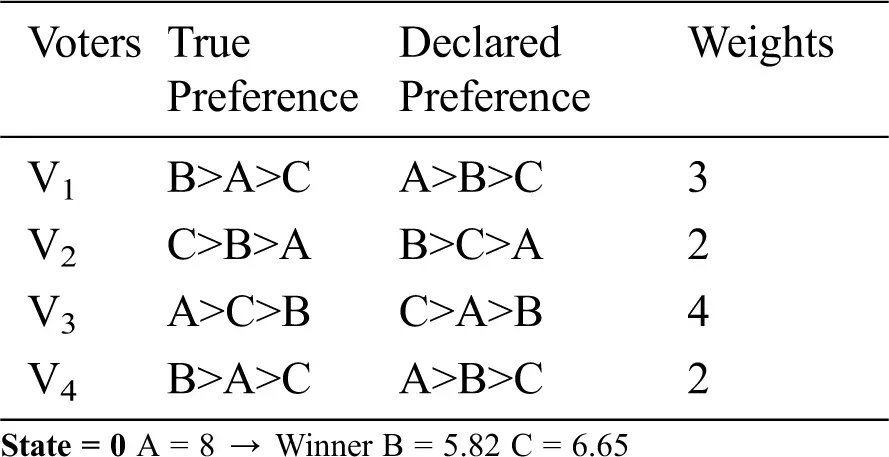Table 5a: State = 0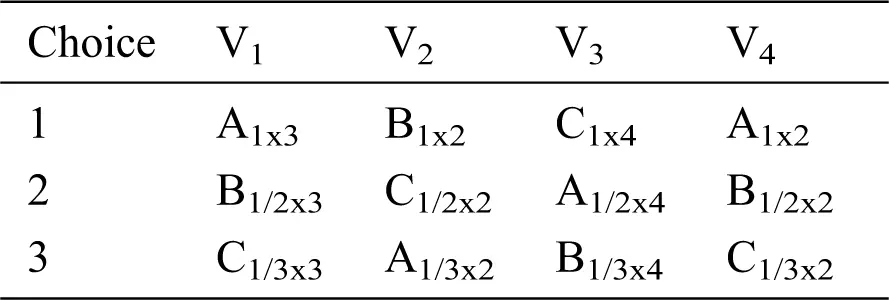In order to get the total score of candidates we multiply the Nauru points to the weights of the voters.

3.18 Problem Statement

In this research we have analysed the dynamics of the Nauru electoral system, allowing voters to choose their favourite candidate in each state and make him their representative. Nauru is the modified version of Borda and is more accurate. In Borda, voters give 0 point for last preference and 1 point for penultimate preference but in Nauru voting system, voters give 1 point for first choice and ½ point for second choice and so on. In this paper, we have used Copeland’s method as a tie rule along with the traditional linear comparison method, i.e., lexicographic method.

In the election, voters are allowed to change their preferences and try to make their favourite candidate the winner of the election. The manipulation dynamics of the election for the Nauru electoral system has been analysed for the first time where the voters have incomplete information, they only know about the current scores of the candidates. We look for the scenarios where convergence is not guaranteed.

3.19 Nauru Weighted Voting System

Example 6 Voters start from their truthful state.

Table 6: Nauru Weighted Point SystemState = 0

True Preference result: In order to get the total score of candidate we multiply the Nauru points to the weights of the voters.

Table 6a: State = 0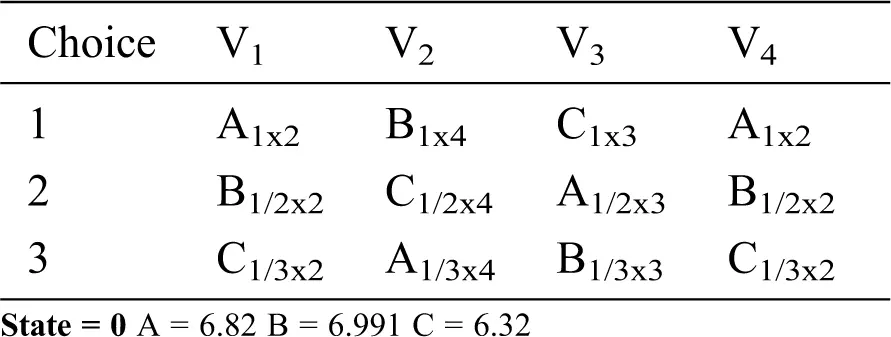Table 6b: State =1 V3: CAB → ACB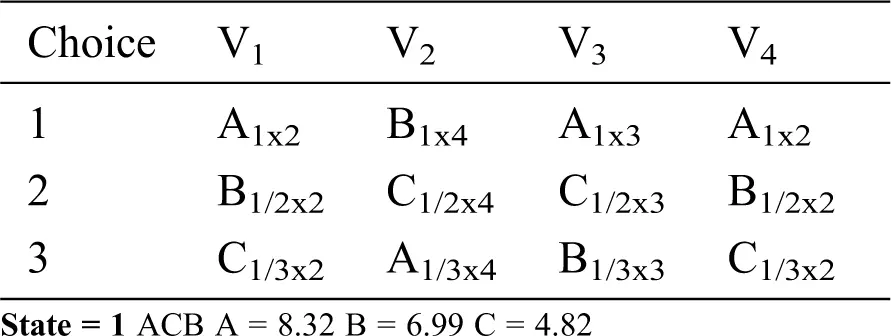In this example candidate B is the winner in State S0 but in state S1 only voter V3 can change his preference from CAB → ACB as a result the outcome of election is changed.

3.20 Example 7

Tie Breaking rules used are Copeland’s method and Lexicographical rule and voters start from their declared preferences.

Table 7: Tie Breaking rules for Nauru weighted point system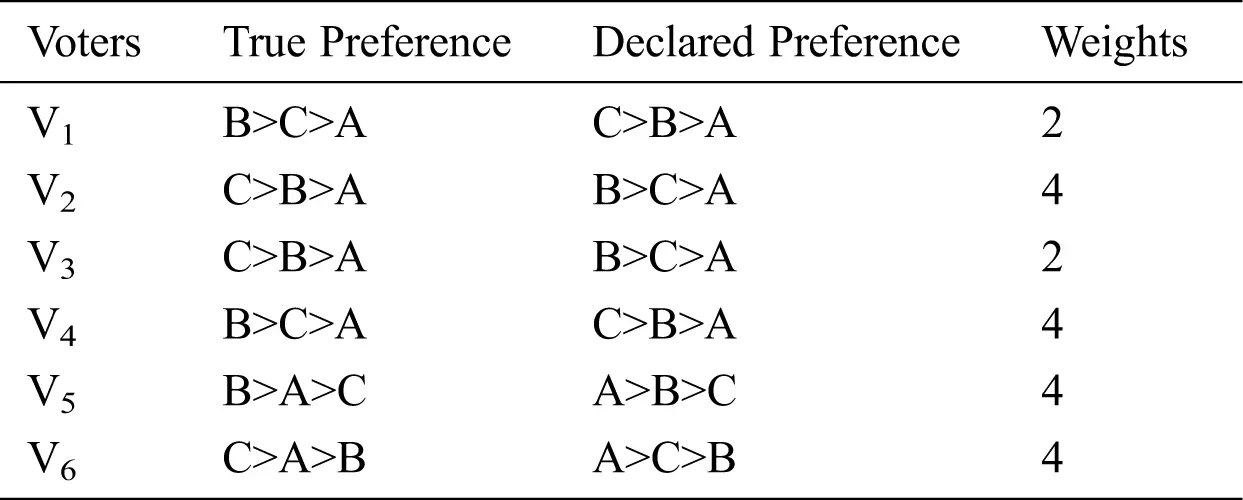State = 0

In order to get the total score of candidate we multiply the Nauru points to the weights of the voters.

Table 7a: State = 0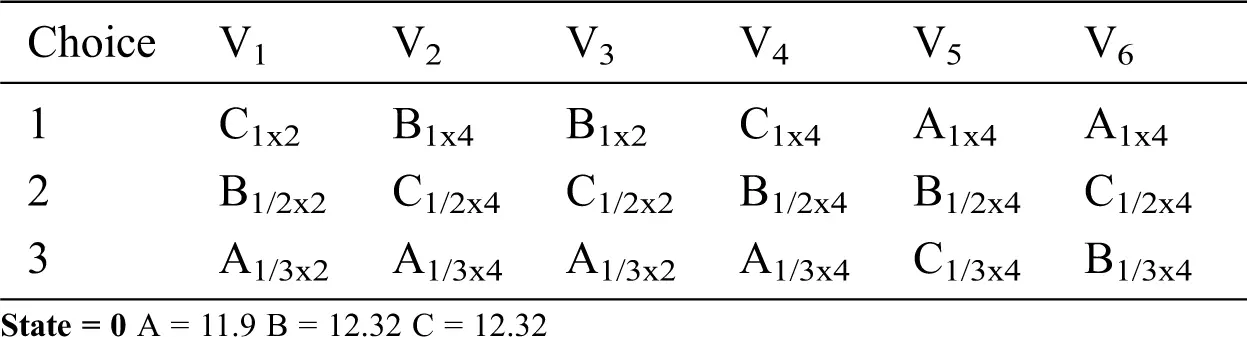According to the lexicographical rule B is the winner of the election.

Table 7b: State = 1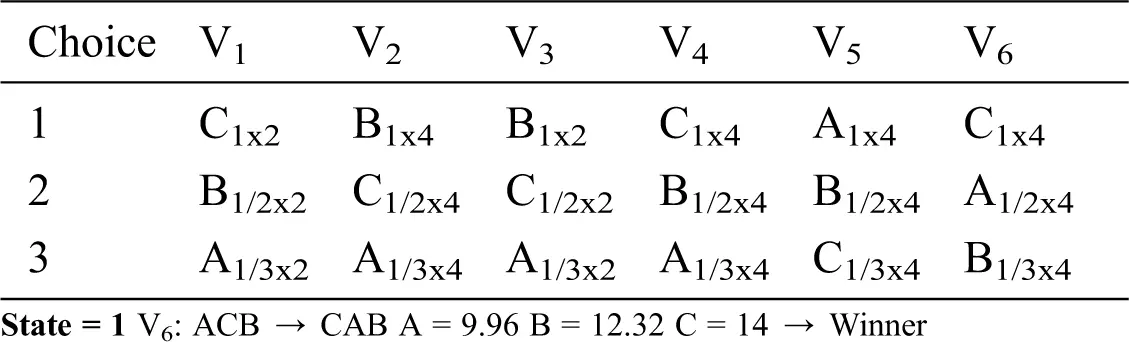In this example candidate B is the winner at State S0 but in state S1 voter V6 changes his preference from ACB → CAB, this transition effects the overall outcome of the election and candidate C becomes winner of state S1.

4  Cycles of Iterative Voting for Nauru Voting System

4.1 Example 8

Let’s voters start from their declared state.

Table 8: Cycles of Iterative Voting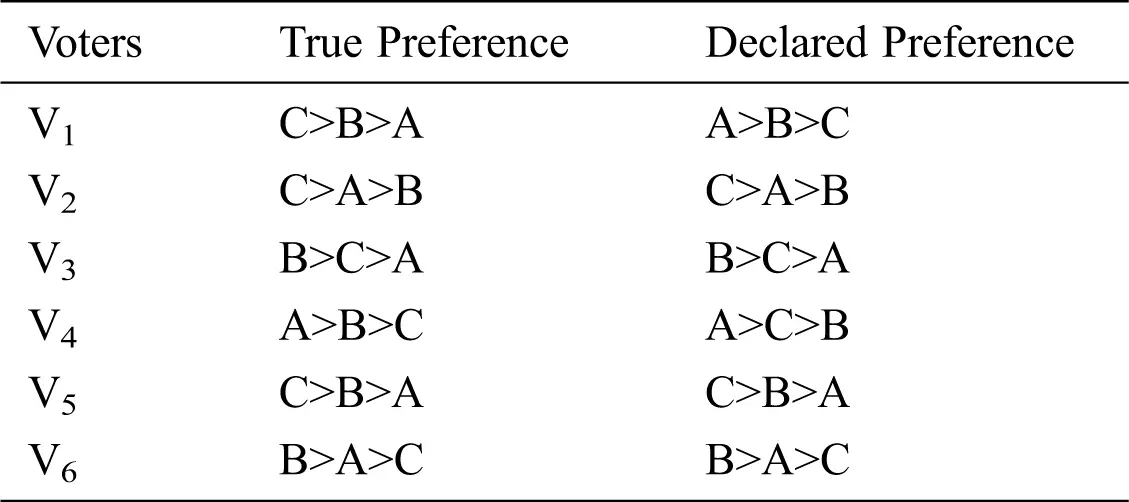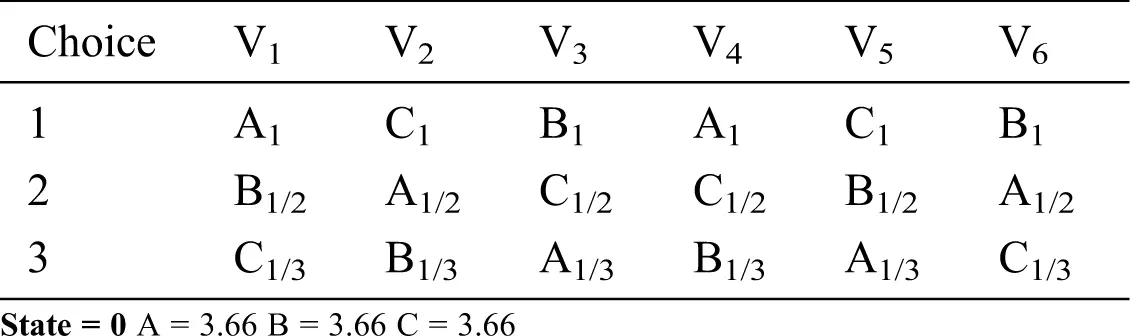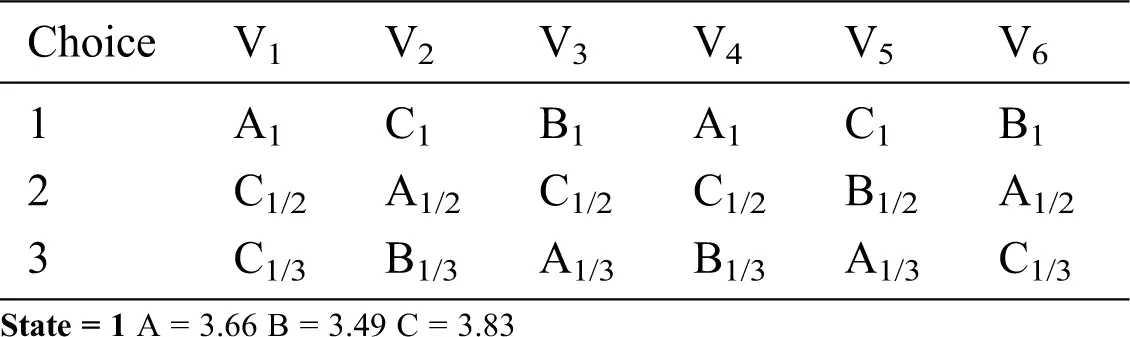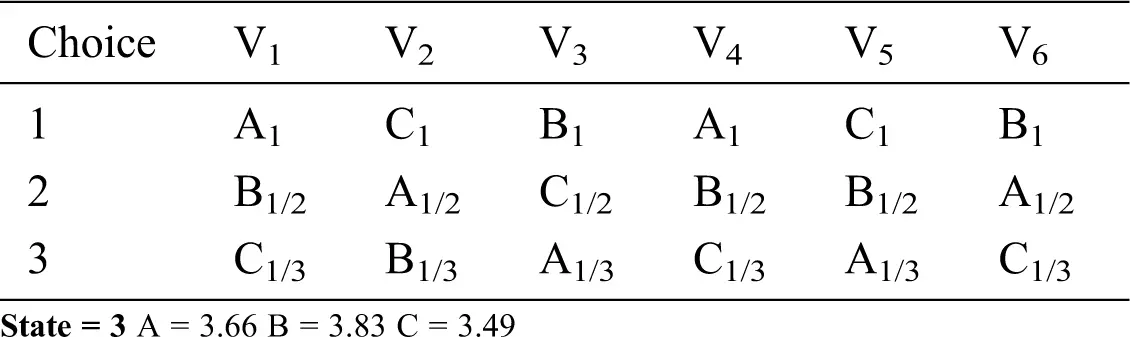The above example shows the cycle for the Nauru voting system when Type 1 and Type 3 moves are considered and when voters start from their stated preferences.

4.2 Example 9

Let’s voters start from their truthful state.

Table 9: Cycle for Nauru Voting System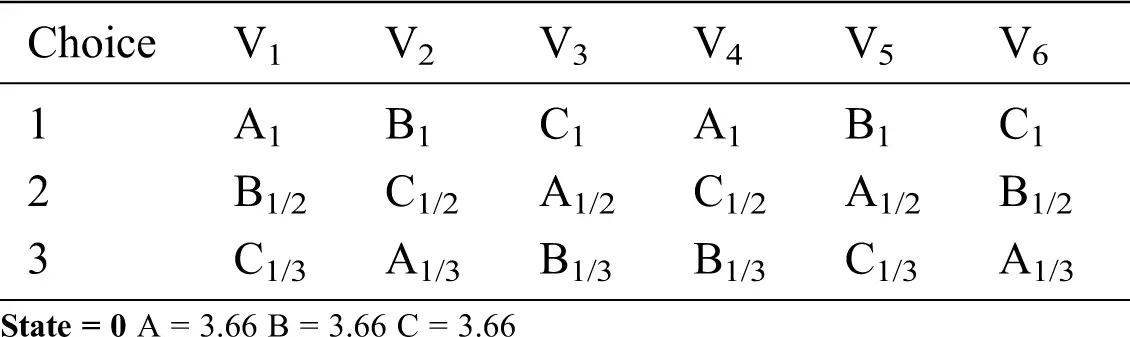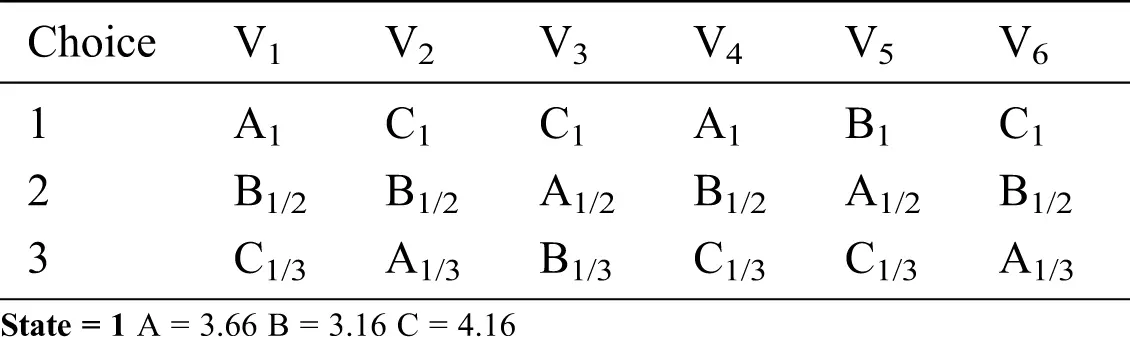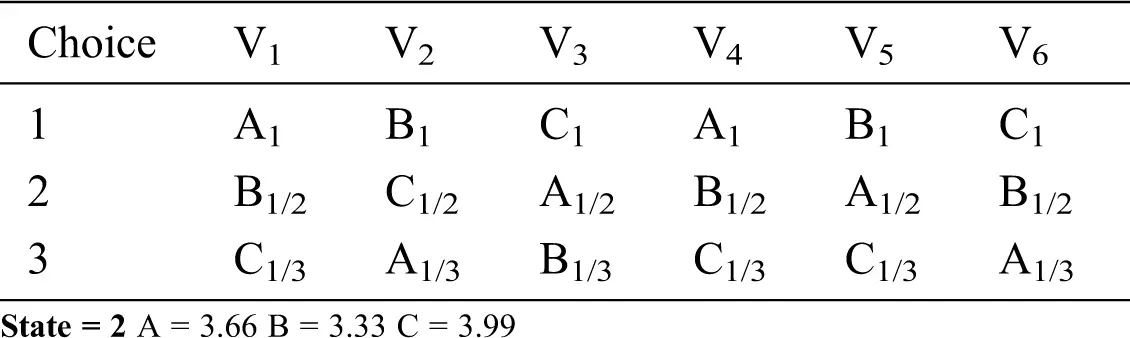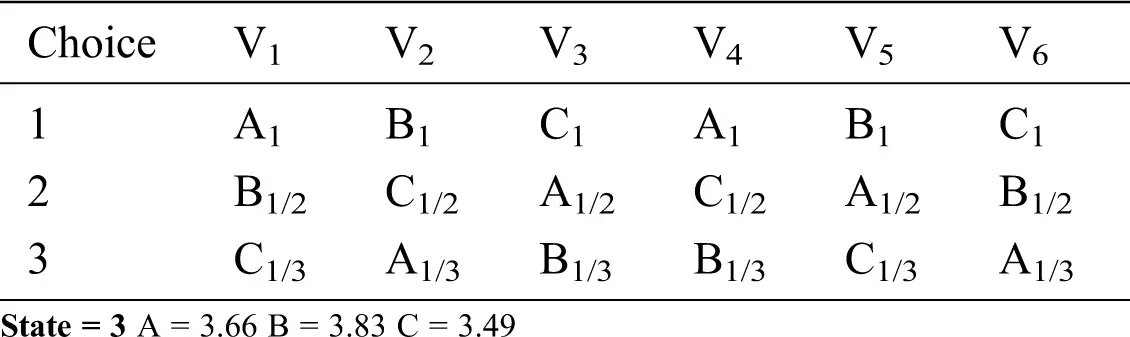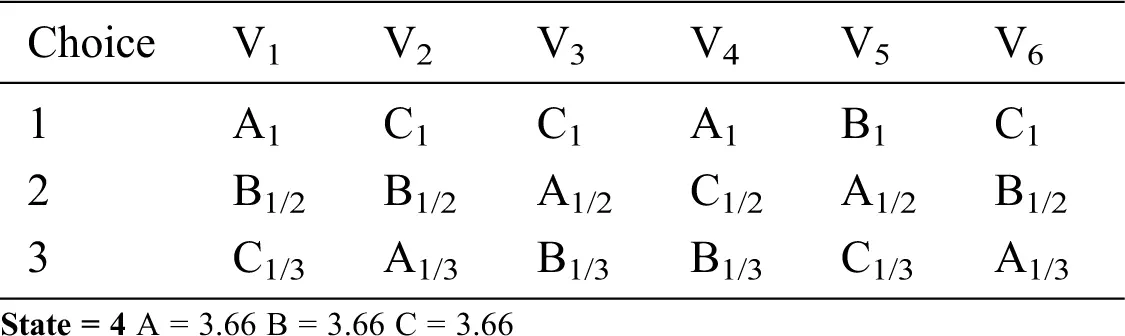The above example shows the non-convergence result for the Nauru voting system when type 1, type 3, and type 4b moves are considered and when voters assume their truthful state.

Example 8 and 9 show that regardless of whether voters assume their truthful or declared state, the iterative voting process for Nauru voting does not converge when these three manipulative moves are used in the unweighted setting. A mechanism is needed to break the cycle and achieve equilibrium.

5  Dynamics for Nauru Weighted Voting System

5.1 Example 10

The moves used in this example are loser-winner, winner-new winner, and winner-existing winner. Voters start with their stated preferences and use the moves above. The following tables show the transition from one state to another by executing a manipulative move in the weighted setting.

Table 10: Nauru Weighted Voting System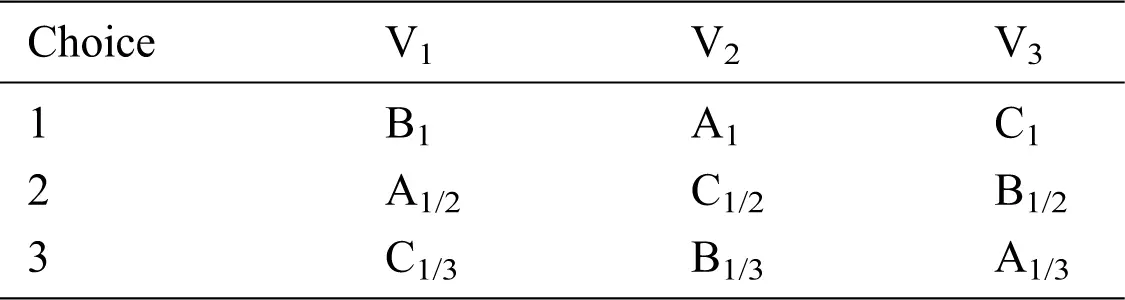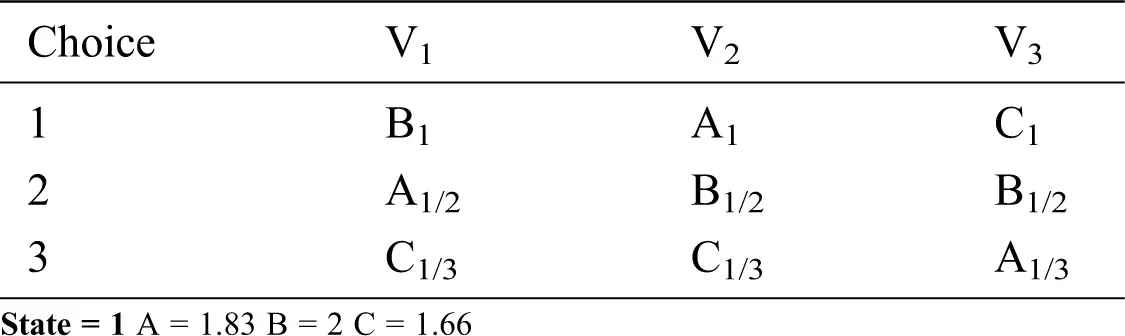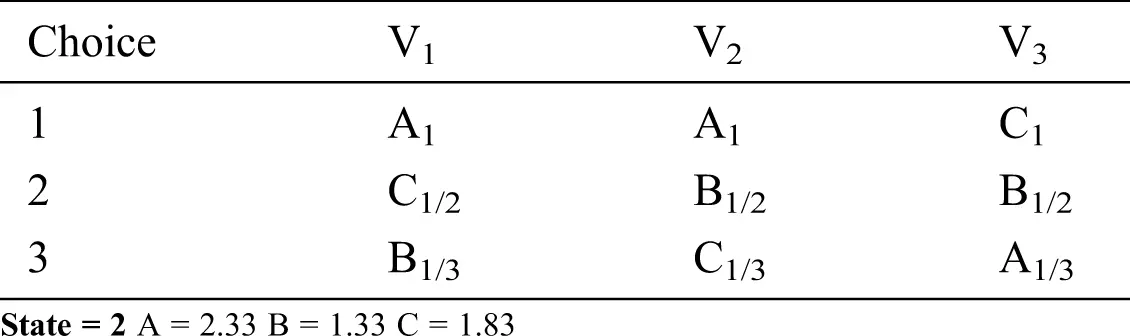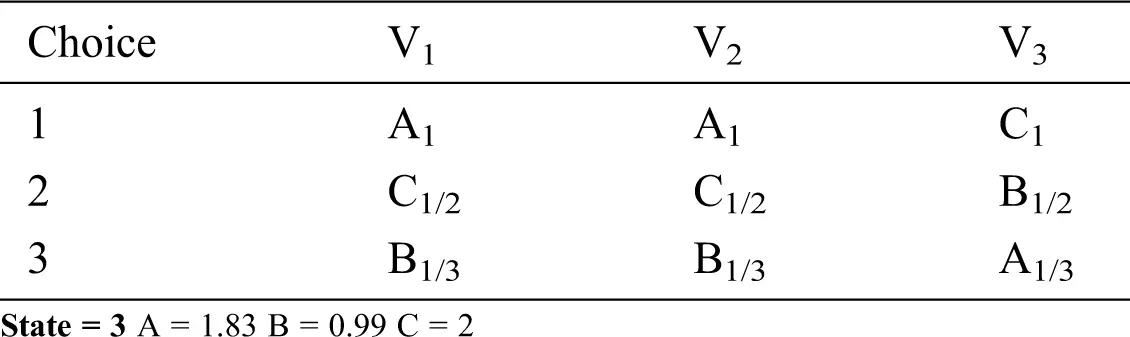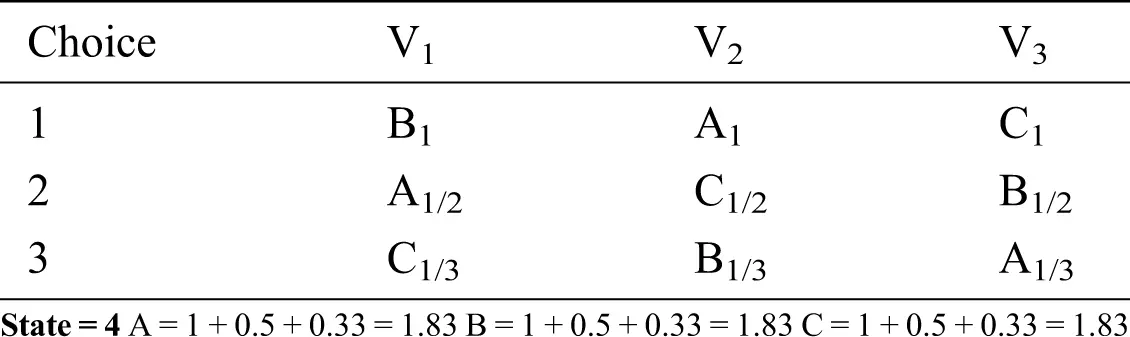Cycle is generated for the given set of moves.

5.2 Example 11

Moves used are loser to winner, winner to new winner and winner to existing winner. Voters start from their declared preference list.

Table 11: Nauru weighted voting system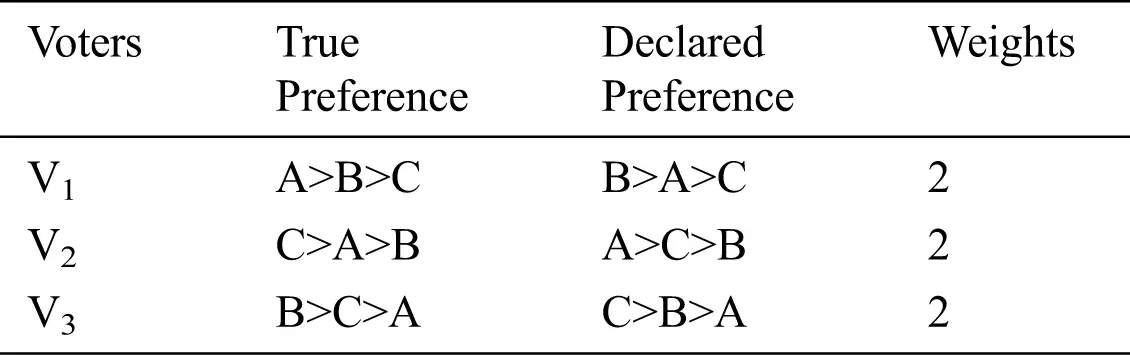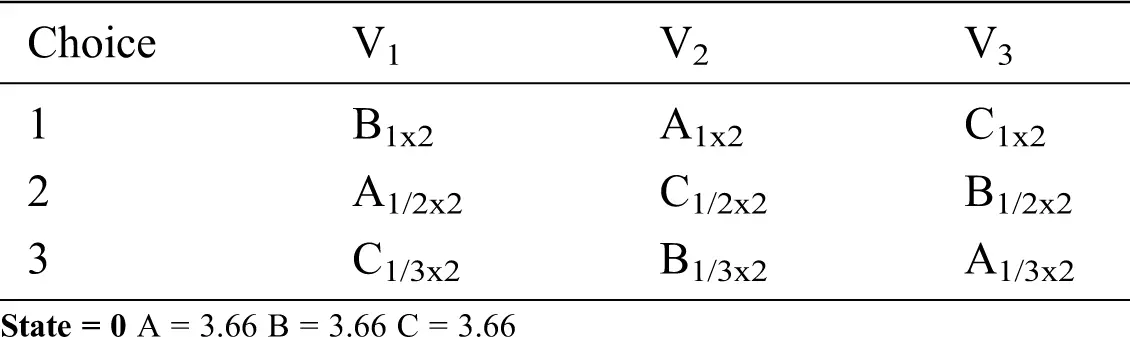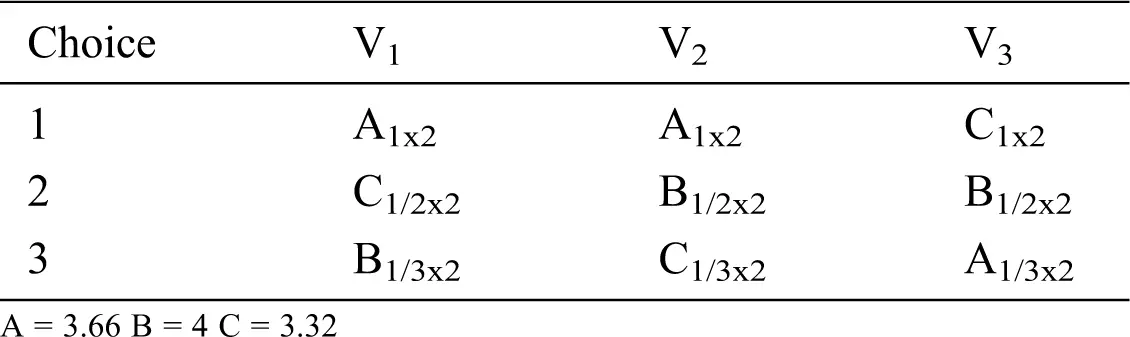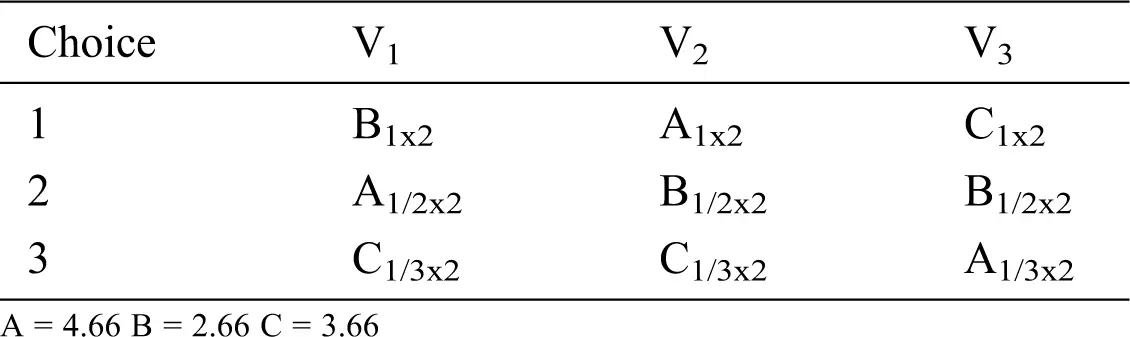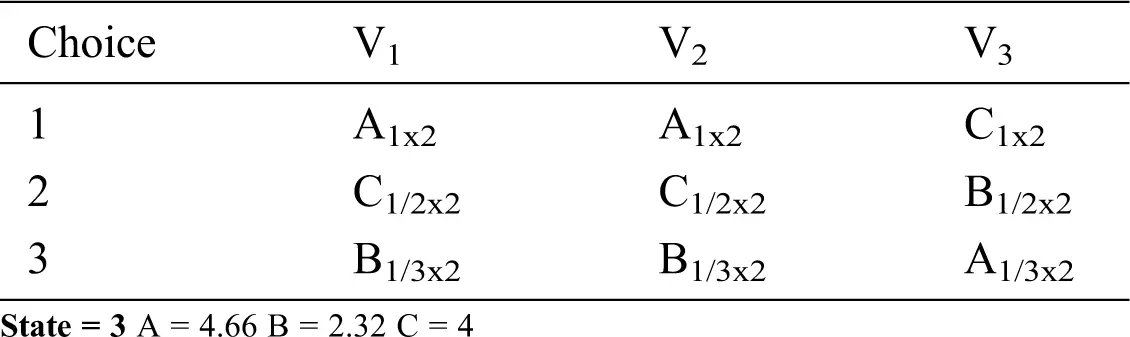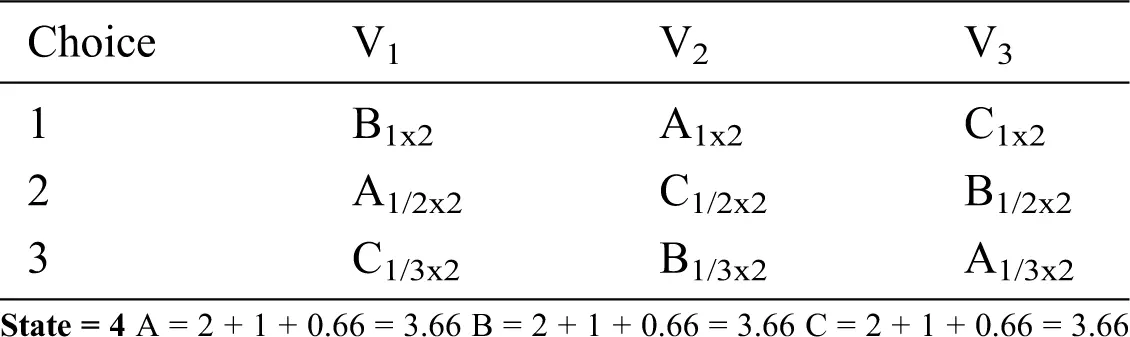5.3 Example 12

Weighted Nauru point system is used and the weights are real numbers. The moves used in this example are loser-winner, winner-new winner, winner-existing winner, and winner-loser.

Table 12: Weighted Nauru point system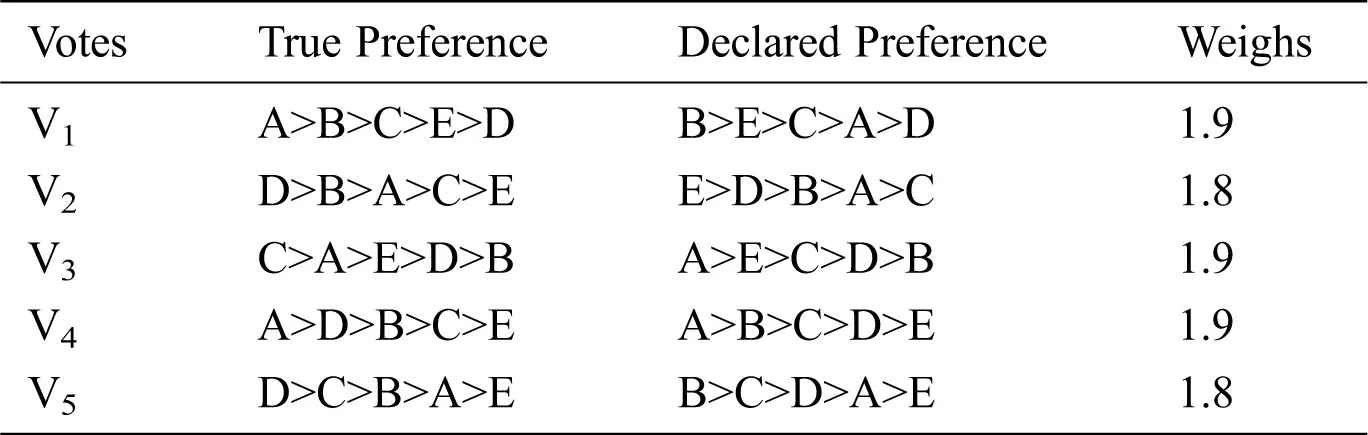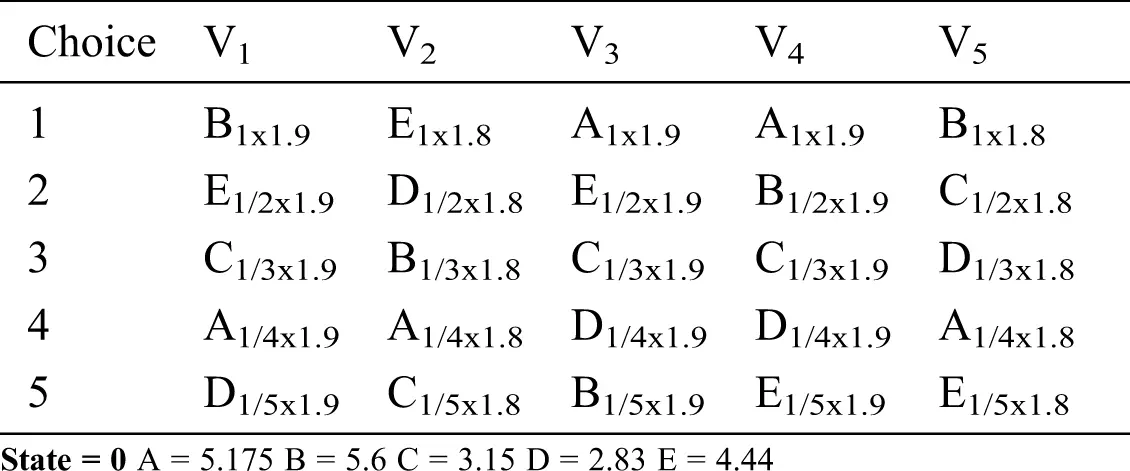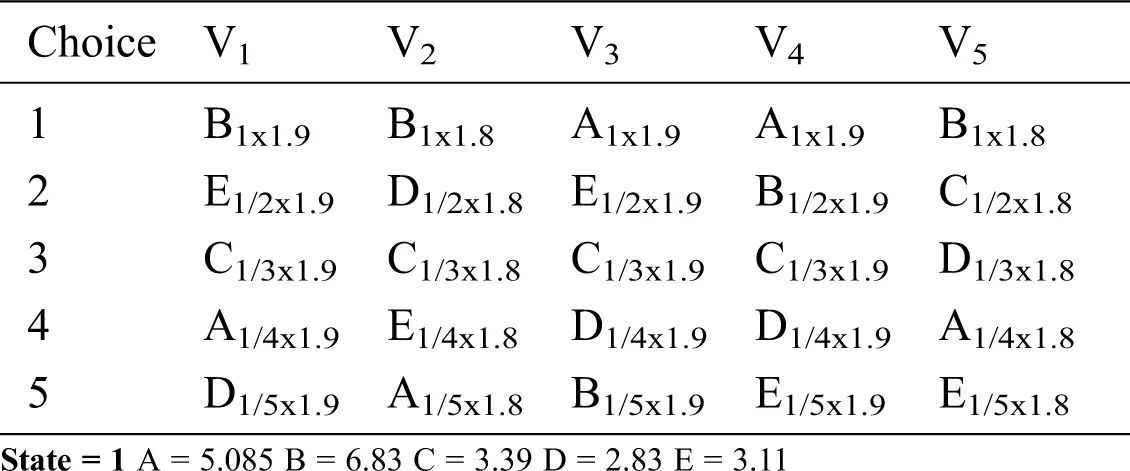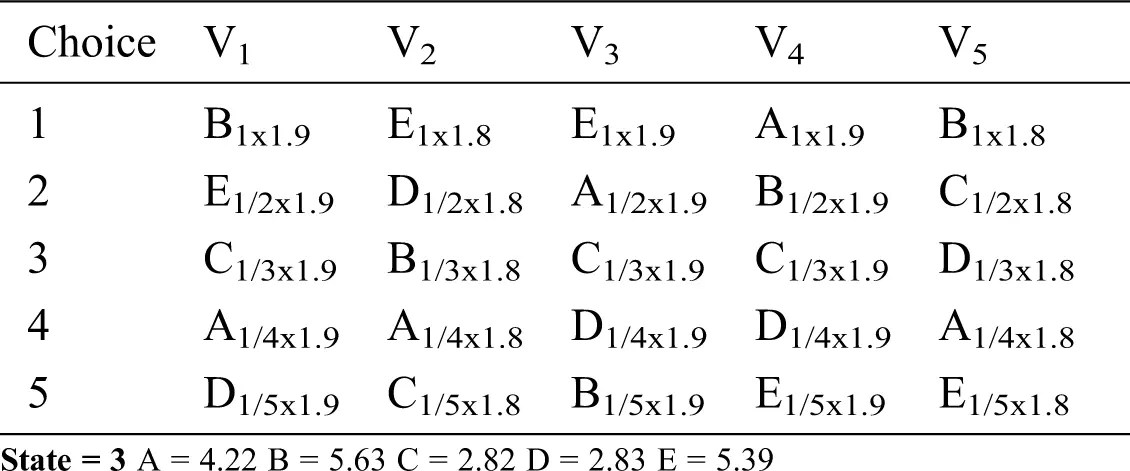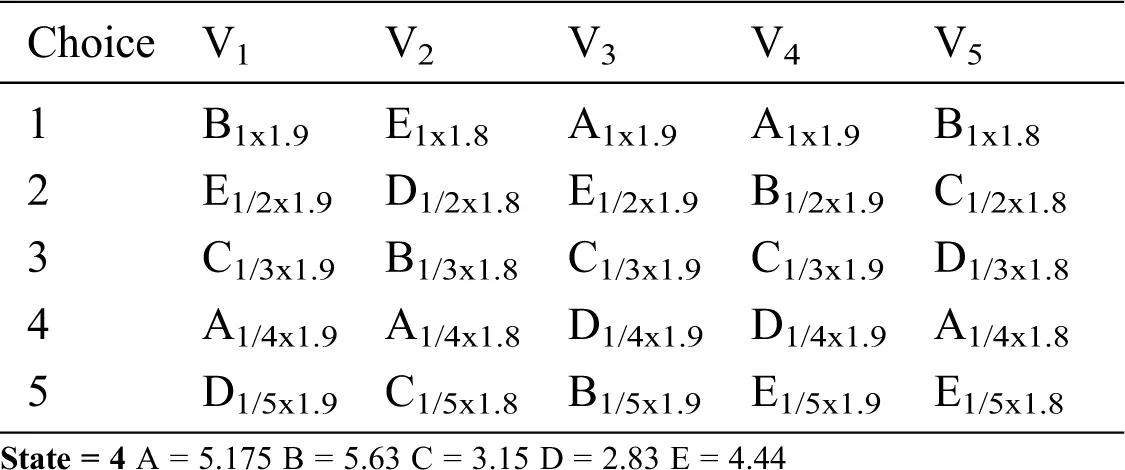5.4 Example 13

Moves = Loser-winner Winner-new Winner Winner –Existing Winner Winner – Loser

Voters start from their declared state

Table 13: Nauru Weighted Voting System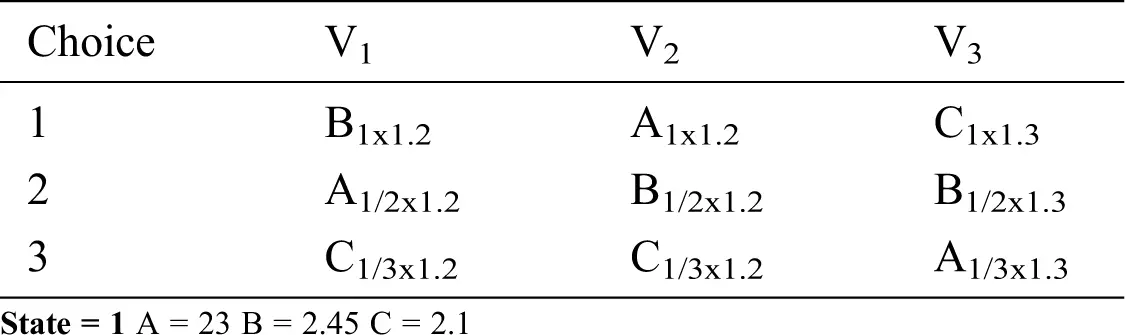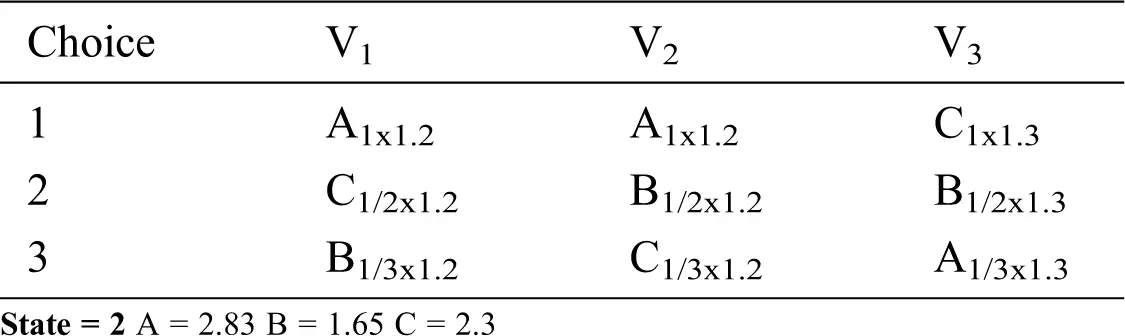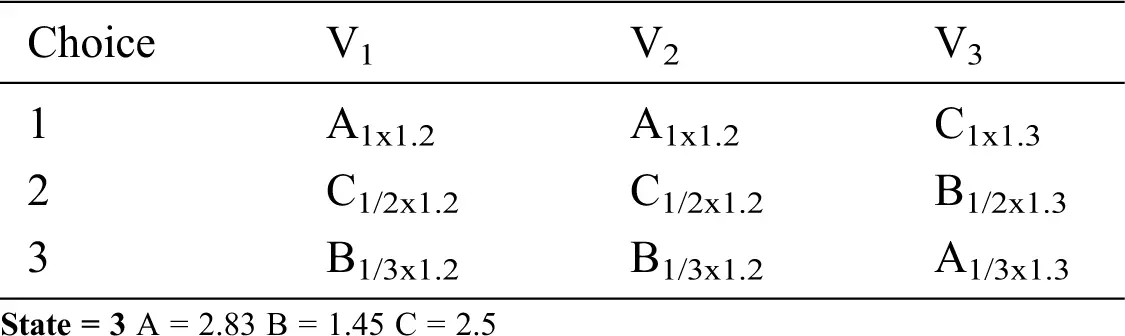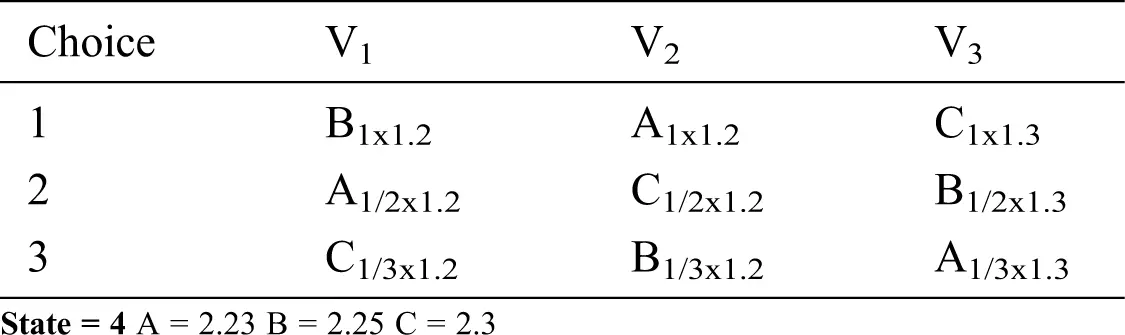Examples 10, 11, 12, and 13 show that the Nauru voting system generates cycles in weighted settings for various subsets of manipulative moves, regardless of whether we consider real or integer weights. Voters assume their stated preference and have incomplete information about the preferences of other voters, in case of ties, equality rules are used and voters choose better and best answers. An interesting direction is how to break the cycle to converge the process of manipulation.

6  Conclusions

The challenge in the electoral system is the issue of manipulation, with some manipulations being NP-hard. As an alternative game-theoretic approach to evaluating the process of preference aggregation, Nash equilibria can be considered as a solution concept for aggregating the preferences of strategic voters.

For the iterative process for Nauru with tie breaking such as lexicographic or Copeland method, cycles are observed for different sets of moves. The iterative process for Nauru was analysed for the first time and we consider that voters have incomplete information about candidate preferences. Our results show that voters may assume a declared or truthful preference, but the process does not converge for a given set of moves. Further analysis can be done for the Nauru voting rule and can be constrained like convergence in the case of best-response dynamics. Randomised tie-breaking can also be applied to Nauru. Other profiles that contain cycles or convergence are of interest for the study of dynamics. In the context of voting systems, it can also be considered as a method for consensus building and can be used in some applications such as Doodle for event scheduling.

This analysis of dynamics helps us to compare and select the relevant voting model based on the properties of Nash Equilibrium or non-convergence. Another direction to explore is the dependence of convergence or non-convergence on various attributes such as tie-breaking rule or weighted settings.

Funding Statement: The authors received no specific funding for this study.

Conflicts of Interest: The authors declare that they have no conflicts of interest to report regarding the present study.

## References

1. W. H. Riker and P. C. Ordeshook. (1968). “A theory of the calculus of voting,” American Political Science Review, vol. 62, no. 1, pp. 25–42.
2. O. Shvetsova. (1998). “Making votes count: Strategic coordination in the world’s electoral systems by Gary W. Cox,” Political Science Quarterly, vol. 113, no. 4, pp. 724–725.
3. T. R. Palfrey. (1998). “Laboratory experiments in political economy,” Annual Review of Political Science, vol. 12, no. 1, pp. 379–388.
4. C. Dwork, R. Kumar, M. Naor and D. Sivakumar. (2001). “Rank aggregation methods for the web,” in Proc. 10th Int. Conf. World Wide Web, pp. 613–622.
5. D. M. Pennock, E. Horvitz, S. Lawrence and C. L. Giles. (2000). “Collaborative filtering by personality diagnosis: A hybrid memory-and model-based approach,” Proc. 16th Conf. Uncertain Artificial Intelligence, vol. 64, no. 10, pp. 473–480, , [Online]. Available: http://citeseerx.ist.psu.edu/viewdoc/download?.
6. L.-M.-U. M. Technische Universtität München. (2018). “Manipulation of voting scheme,” e-conversion—Proposal for a Cluster of Excellence, vol. 41, no. 4, pp. 587–601.
7. M. A. Satterthwaite. (1975). “Strategy-proofness and arrow’s conditions: existence and correspondence theorems for voting procedures and social welfare functions,” Journal of Economic Theory, vol. 10, no. 2, pp. 187–21
8. R. Meir, M. Polukarov, J. S. Rosenschein and N. R. Jennings. (2010). “Convergence to equilibria in Plurality voting,” Proc. of National Conf. Artificial Intelligence, vol. 2, pp. 823–82
9. N. Immorlica, L. (Erran) Li, V. S. Mirrokni and A. S. Schulz. (2009). “Coordination mechanisms for selfish scheduling,” Theoretical Computer Science, vol. 410, no. 17, pp. 1589–1598.
10. N. Gohar and P. W. Goldberg. (2013). “Potential functions for voting dynamics,” in Proc. On The Int. Conf. on Artificial Inteligence (ICAIThe Streeting Committee of the World Congress in Computer Science, Computer Engineering and Applied Computing (World Computing).
11. A. Reijngoud and U. Endriss. (2012). “Voter response to iterated poll information,” 11th Int. Conf. on Autonomous Multiagents System 2012, AAMAS, vol. 1, no. Illc, pp. 376–383.
12. N. Gohar. (2017). Manipulative Voting Dynamics. Newcastle upon Tyne, UK: Cambridge Scholar Publishing.
13. N. Gohar, S. Noor, F. F. Babar, A. Malik and S. Shaheen. (2019). “Dynamics of manipulation in voting, veto and plurality,” Cluster Computing, vol. 22, no. S3, pp. 7333–7345.
14. E. Ephrati and J. S. Rosenschein. (1996). “Deriving consensus in multiagent systems,” Artificial Intelligence, vol. 87, no. 1–2, pp. 21–74.
15. S. Airiau and U. Endriss. (2009). “Iterated majority voting,” in Lecture Notes Computer Science (Including Subseries Lecture Notes Artificial Intelligence Lecture Notes Bioinformatics), vol. 5783 LNAI, pp. 38–49.
16. J. J. Laffont. (1987). “Chapter 10 Incentives and the allocation of public goods,” Handbook of Public Economics, vol. 2, pp. 537–569.
17. T. J. Feddersen, I. Sened and S. G. Wright. (1990). “Rational voting and candidate entry under plurality Rule,” American Journal of Political Science, vol. 34, no. 4, pp. 1005.
18. M. Messner and M. K. Polborn. (2007). “Strong and coalition-proof political equilibria under plurality and runoff rule,” Int. Journal of Game Theory, vol. 35, no. 2, pp. 287–314.
19. S. Chopra, E. Pacuit and R. Parikh. (2004). “Knowledge-theoretic properties of strategic voting,” Lecture Notes Artificial Intelligence (Subseries Lecture Notes Comput. Science, vol. 3229, pp. 18–30.
20. R. B. Myerson and R. J. Weber. (1993). “A theory of voting equilibria,” American Political Science Review, vol. 87, no. 1, pp. 102–114.
21. O. Lev and J. S. Rosenschein. (2012). “Convergence of iterative voting,” 11th Int. Innovative Conf. Autonomous Agents Multiagent System 2012, AAMAS 2012 Innovation Application Track, vol. 1, pp. 352–359.
22. A. P. Sage. (1988). “Group decision making under multiple criteria: Methods and applications,” European Journal of Operational Research, vol. 33, no. 1, pp. 132.
23. P. Dalapati, P. Agarwal, A. Dutta and S. Bhattacharya. (2018). “Dynamic process scheduling and resource allocation in distributed environment: An agent-based modelling and simulation, Mathematical and,” Computing Modeling of Dynamic System, vol. 24, no. 5, pp. 485–505.
24. D. R. M. Thompson, O. Lev, K. Leyton-Brown and J. Rosenschein. (2013). “Empirical analysis of plurality election equilibria,” in 12th Int. Conf. on Autonomous Agents Multiagent System 2013, AAMAS. vol. 1, pp. 391–398.
25. L. Dery, S. Obraztsova, Z. Rabinovich and M. Kalech. (2019). “Lie on the fly: Strategic voting in an iterative preference elicitation process,” Group Decision and Negotiation, vol. 28, no. 6, pp. 1077–1107.
26. R. Meir, M. Polukarov, J. S. Rosenschein and N. R. Jennings. (2017). “Iterative voting and acyclic games,” Artificial Intelligence, vol. 252, no. 1, pp. 100–122.
27. R. Reyhani and M. C. Wilson. (2012). “Best reply dynamics for scoring rules,” Frontiers Artificial Intelligence Application, vol. 242, no. October, pp. 672–677.
28. L. Xia, M. Zuckerman, A. D. Procaccia, V. Conitzer and J. S. Rosenschein. (2009). “Complexity of unweighted coalitional manipulation under some common voting rules,” IJCAI Int. Joint Conf. on Artificial Intelligence, vol. 9, pp. 348–353.
29. R. Meir. (2016). “Strong and weak acyclicity in iterative voting,” in Lecture Notes Computer Science (Including Subseries Lecture Notes Artificial Intelligence Lecture Notes Bioinformatics). vol. 9928 LNCS, pp. 182–194.
30. N. S. Kukushkin. (2011). “Acyclicity of improvements in finite game forms,” Int. Joint Conf. of Game Theory, vol. 40, no. 1, pp. 147–177.This work is licensed under a Creative Commons Attribution 4.0 International License, which permits unrestricted use, distribution, and reproduction in any medium, provided the original work is properly cited.